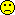## FDA group model in R [Two-Stage / GS Designs]

Dear zizou,

Thank you for suggestion!
The FDA group model for PHX is described by Helmut here:
fixed:  Group+Sequence+Sequence(Group)+Period(Group)+Treatment+Treatment×Group random: Subject(Sequence×Group)

I think in this case PHX easily switches from GLM to MIXED procedure and does not produce SS tables.
We all know that the GLM procedure should be used for conventional FDA model. Could we use the same procedure (GLM) here?

The relationship between GLM/MIXED is described very well in 'must-read' post by ElMaestro

I executed the code with unequal sequences (removing Subj18 from the reference dataset):
PHX Sequential tests:
  Dependent Hypothesis Numer_DF Denom_DF        F_stat     P_value 1   Ln(Var)        int        1       13 2830.51538137 0.000000000 2   Ln(Var)      Group        1       13    1.01359006 0.332416756 3   Ln(Var)        Seq        1       13    0.69165428 0.420616500 4   Ln(Var)  Group*Seq        1       13    0.12190952 0.732563224 5   Ln(Var)  Group*Per        2       13    2.99449184 0.085186463 6   Ln(Var)        Trt        1       13    3.42927592 0.086890932 7   Ln(Var)  Trt*Group        1       13    0.34285051 0.568214408

PHX Partial tests:
  Dependent Hypothesis Numer_DF Denom_DF        F_stat     P_value 1   Ln(Var)        int        1       13 2803.64675463 0.000000000 2   Ln(Var)      Group        1       13    1.14134985 0.304804606 3   Ln(Var)        Seq        1       13    0.65955233 0.431338627 4   Ln(Var)  Group*Seq        1       13    0.12190952 0.732563224 5   Ln(Var)  Group*Per        2       13    3.18289277 0.074972760 6   Ln(Var)        Trt        1       13    3.53470601 0.082691107 7   Ln(Var)  Trt*Group        1       13    0.34285051 0.568214408

R:
muddle <- lm(log(Var)~Group+Seq+Seq*Group+Subj%in%(Seq)+Per%in%Group+Trt+Group*Trt, data=data) anova(muddle)
              Df  Sum Sq   Mean Sq  F value     Pr(>F)    Group           1 0.30128 0.3012782 40.66580 2.4299e-05 *** Seq             1 0.20559 0.2055864 27.74955 0.00015211 *** Trt             1 0.02101 0.0210135  2.83635 0.11599640    Group:Seq       1 0.03624 0.0362362  4.89108 0.04551863 *  Group:Per       2 0.04876 0.0243815  3.29096 0.06975471 .  Group:Trt       1 0.00254 0.0025401  0.34285 0.56821441    Group:Seq:Subj 13 3.86410 0.2972387 40.12055 2.5513e-08 *** Residuals      13 0.09631 0.0074086 

drop1(muddle,test="F")
 Single term deletions Model: log(Var) ~ Group + Seq + Seq * Group + Subj %in% (Seq * Group) +     Per %in% Group + Trt + Group * Trt                Df Sum of Sq     RSS       AIC  F value     Pr(>F)    <none>                      0.09631 -157.4617                        Group:Per       2   0.04716 0.14347 -147.9107  3.18289   0.074973 .  Group:Trt       1   0.00254 0.09885 -158.5766  0.34285   0.568214    Group:Seq:Subj 13   3.86410 3.96042  -57.1004 40.12055 2.5513e-08 ***              
and CI's are far from equality...PS: I tried to switch to the mixed model
library(nlme) Group222FDA<- function(data, alpha=0.05) {   data$Per <- factor(data$Per)  # Period   data$Seq <- factor(data$Seq)  # Sequence   data$Trt <- factor(data$Trt)  # Treatment   data$Subj <- factor(data$Subj) # Subject   data$Group <- factor(data$Group)     muddlelme <- lme(log(Var) ~ Group + Seq + Seq %in% Group                    + Per %in% Group + Trt +  Trt * Group ,                    random=~1|Subj, data=data, method="REML")   lnPE     <- coef(muddlelme)[1, "TrtT"]   lnCI     <- confint(muddlelme,c("TrtT"), level=1-2*alpha)[][1:2]   PE <- exp(lnPE)   CI <- exp(lnCI)   typeI <- anova(muddlelme)   # output   options(digits=8)   cat("Type I sum of squares: \n")   print(typeI)   cat("\nBack-transformed PE and ",100*(1-2*alpha))   cat("% confidence interval\n")   cat("Point estimate (GMR).(%).................:",       formatC(100*PE, format="f", digits=2),"\n")   cat("Lower confidence limit.(%)...............:",       formatC(100*CI, format="f", digits=2) ,"\n")   cat("Upper confidence limit.(%)...............:",       formatC(100*CI,format="f", digits=2) ,"\n") } data <- read.delim("https://static-content.springer.com/esm/art%3A10.1208%2Fs12248-014-9661-0/MediaObjects/12248_2014_9661_MOESM1_ESM.txt") Group1 <- subset(data, Subj <= 9) Group1$Group <- 1 Group2 <- subset(data, Subj > 9& Subj < 18) Group2$Group <- 2 data<-rbind(Group1, Group2) Group222FDA(data, alpha=0.05)
The ANOVA type I is the same as in PHX, but PE and CIs are not. I'm in stuck....

Kind regards,
MittyriIng. Helmut Schütz